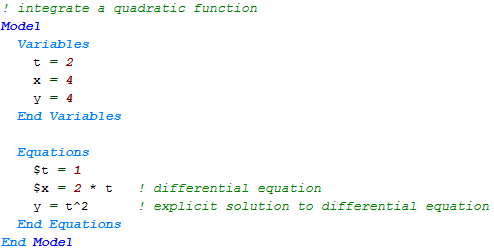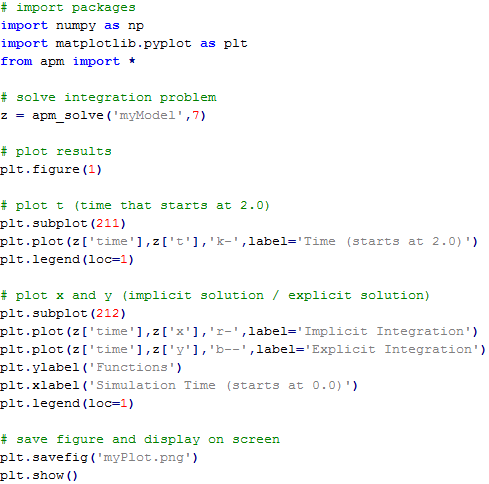## Tutorial Examples

Models presented below are a test suite of optimization problems. They are part of the test suite used to verify modifications to the APMonitor software. Users may also find this collection useful to compare APMonitor syntax to other modeling languages, for solver benchmarking, or as example problems in optimization. Models may be submitted through the Online Web-based Interface.

#### Test problem with 2 local minima

This optimization problem has 2 local minima. The two problem solutions are found at (0,0,8) with objective 936.0 (global minimum) and (7,0,0) with objective 951.0.#### Numerical Integration

This problem demonstrates how to numerically integrate a function. In this case, the function of interest is x = 2 t with an exact solution of y = t2 .The following Python script runs the model and plots the results: